Reach Us+44-1522-440391
Effects of Causality and Error Correction on Volatility Modeling: A Simulation Approach | OMICS International
International Journal of Economics & Management Sciences
All submissions of the EM system will be redirected to Online Manuscript Submission System. Authors are requested to submit articles directly to Online Manuscript Submission System of respective journal.

# Effects of Causality and Error Correction on Volatility Modeling: A Simulation Approach

Rotich Titus Kipkoech*

Student, Mount Kenya University , Eldoret, Kenya

*Corresponding Author:
Rotich Titus Kipkoech
Student, Mount Kenya University
Eldoret-9305-30100, Kenya
Tel: (053) 2033712
E-mail: [email protected]

Received date: October 04, 2015 Accepted date: October 23, 2015 Published date: October 28, 2015

Citation: Kipkoech RT (2015) Effects of Causality and Error Correction on Volatility Modeling: A Simulation Approach. Int J Econ Manag Sci 4:297. doi:10.4172/2162-6359.1000297

Copyright: © 2015 Kipkoech RT. This is an open-access article distributed under the terms of the Creative Commons Attribution License, which permits unrestricted use, distribution, and reproduction in any medium, provided the original author and source are credited.

Visit for more related articles at International Journal of Economics & Management Sciences

#### Abstract

Generalized Auto Regressive Conditional Heteroskedasticity (GARCH) models are usually used to analyse time series data with high volatility clustering. In this paper, we analyse the effects of Granger Causality Model (GCM) and Error Correction Model (ECM) in analysing a time series and accordingly, we simulate two series of data using the GARCH1 model which are used for the analysis. The choice of the simulation model is based on its ability to capture volatility and heteroskedasticity. GCM2 and ECM3 models’ parameters are investigated for adequacy. Results from Augmented Dickey Fuller (ADF), Phillips PerronPhillips Perron (PP) and Kwiatkowski Philips Schmidt Shin (KPSS) tests indicate stationarity in the data as expected. GCM is built to demonstrate all the long term relationships. The two series Granger Caused each other. A linear ECM is also fitted and there is evidence that a short-term relationship exists between these two series. A high threshold value exists at the second lag, an indication of simple smoothing in the data. The residual deviance was greater than the degrees of freedom asserting that the model perfectly fit the data, supported by high R2 value of 0.871. Residuals from the fitted linear model are also stationary. The study concludes that ECMs and GCMs are appropriate in analysing time series. It is recommended that a similar study be undertaken but with a combination of ARMA Auto Regressive Moving Average (ARMA) Process and GARCH models. Further study should also be conducted on tail clustering analysis.

#### Keywords

Granger causality, Error correction model, Simulation, Economics, Volatility

#### JEL Classification

60K35, Granger causality, Error correction model, Simulation, Economics, Volatility

#### Introduction

Escalation of interest rates and exchange rates in Kenya have been a common phenomenon. Its random inter-data movements leads to the subject of volatility. It has formed the basis of most researches in time series analysis, with several scholars building various models in the attempt to exhaustively examine the sources of these volatilities and its predictions. Unfortunately, no particular scholar has been able to exhaustively determine the sources of volatilities, neither have they settled on any particular optimum model. Nevertheless, Leykam , Zhongjian , Musyoki et al. , amongst others, concur that modelling of these volatilities are vital for the health of an economy. It has been pointed out that the most important aspect in any volatility analysis, including others, are to be able to; predict the future volatility behaviour4, and atleast establish the risk attached to these movements.

Volatility can be defined as the periodic displacement of a time series from its long-term mean-level. Forces that displace these time series from its mean-level is of great importance. The displacements as well occur in phases, as suggested by the definition; the short term and the long term. Most scholars have not yet done concurrent short term and long term analysis. However, there is a great need to analyze these shocks in two phases, the short and long-term, in order to capture the movements exhaustively; where imputation of the two relationships can be analyzed. Granger  first proposed a procedure, called granger causality, which analyses the long-term movements. On the other hand, an ECM, introduced by Granger in his work which analyses a short-term relation in existence. He was analyzing a balance in an ECM where he realized that there was an imbalance in I(0) Integration of Order 0 or stationarity5 and I(1) Integration of Order 16 series, (Granger).

Earlier, Fung and Hsieh  had used co-integration on their study on hedge funds. They criticized the conventional approaches of model constructions for asset-class indices to be applied in hedging. Seven factors were identified from which a model was built. On analysis of parameter stability, Fung and Hsieh applies the cumulative recursive residual method and plots on a time scale to investigate the reversion of the model parameter in the risk factor model. The factors are cointegrated and hence influence each others’ performance. Fung and Hsieh finally proposed a seven factor model to be applied for hedging.

Later, Leykam  in his work on cointegration and volatility in the European natural gas spot markets tests the Granger causality in volatily markets. Four markets were identified from which spot prices were obtained. Granger causality tests were done for different pairs of the markets. Of the four markets, one (Bunde) market indicated no association with the other three markets. All the hypotheses that the other three markets can be used in predicting volatility in Bunde were rejected at 1% significance level. This meant that Bunde could not be considered a price setter in the European gas spot market.

The study by Fung and Hsieh was further extrapolated by Zhongjian who analyzed the same hedge funds in view of further examining the validity of the method used in deriving the seven factors which had been suggested by Fung and Hsieh for the inclusion in an hedging portfolio. In his research, he highlights that Fung and Hsieh did not provide enough evidence to proof that the procedure used in choosing the factors is quite different from the Sharpe and Fama-French which only relies on one characteristics of the entire market. Contrary to Fung and Hsieh, Zhongjian bases his parameter stability on the adjusted R2 statistic. Zhongjian does not mention the reason for his selection of R2 statistic instead of the cumulative recursive residual. He identifies nine hedge indices which can be included in the hedging strategy. A full rank co-integration in the industry was as well established, and an eight factor model to be used for hedging strategies as the most powerful model, is proposed.

Initially, Rashid  had applied granger causality in agriculture in a study on spatial integration of maize markets undertaken in the post-liberalized Uganda. Different markets were identified. Causality tests were conducated on different pairs of markets, and the results indicated that all pairs which included Kampala and Jinja failed to reject the causality null. This was an indication of a uni-directional causality, implying that the regional maize prices Granger caused the prices in these two large cities. Also, a two directional causality effect was established between Mbale and Hoima indicating a dependence behavior; that is, all deviances in one market affects the other.

Huang and Neftci  investigated co-integration relationship that existed between the swap spreads and various rates such as the LIBOR London Interbank Offered Rate7 rates, US corporate credit spreads and the treasury yield curve; which found evidence of co-integration existence. In their study, they showed that under the ECM framework, the daily swap spreads reacted to the corrective long-run forces except from the short-term fluctuations in the variables. They concluded that the swap spread had a negative effect only on one measure, the treasury yield curve, but positive in all the other rates.

Later on, Petrov  applies ECM in evaluating a pairwise cointegration strategy between the South African equity market and other emerging and developed markets; using the price indices rather than MSCI Morgan Stanley Capital International8 index, as used by Biekpe and Adjasi. There are two reasons; one, that price index is raw and two, it enabled comparisons across different markets. Petrov shows that all the markets was responding slowly to any long term disequilibrium. The integration proved to be high between most markets and hence portfolio selection was the most sensitive task to undertake. Petrov applies an ECM to analyze different portfolios of different sizes . He finds out that USA dominated in all the portfolios in which it was introduced. It was then recommended that such portfolios should be considered the most favorable for investors.

Credit risk is one of the most important type of risk which a bank will be keen to assess to ensure that it remains in business. On the other hand, most of the bank advances are made on a collateral basis. Karumba and Wafula  studies this collateral lending characteristic of the lending institutions9 and their implication on the general financial equilibrium. They investigated its implication on the level of credit risk faced by the banks. Karumba and Wafula applies co-integration and error correction techniques to investigate long-run relationship. The study found over-reliance on collateral in institutional lending. A negative ECM adjustment coefficient was found indicating that advances in loans and collaterals had a short-term adjustment. With the introduction of credit referencing, the study concludes a general reduction in credit risk.

In Basel III accord, the main challenge is addressing rates volatility. Its evolution over time makes credit risk analysis more complex. In this research, we contribute to the bank of literature by investigating existence of short-term and long-term relation between lending and interest rates. This contributes to mitigation of credit risk analyzed by Karumba and Wafula. A procedure for modelling interbank lending rates can be seen as a milestone to the mitigation strategy. It will make the work proposed in Basel III accord much easier.

#### Some Theoretical Review

In this section, we review some important definitions on the various tests which will be used in section (5) for analyses. We start by reviewing definitions on granger causality in sub-section (4.1); followed by a review on ECM in sub-section (2.2), and finally defining some tests in sub-section (4.3), which are fundamental in the study.

Granger causality

In this section, we review basic concepts on granger causality. We define what is granger causality in sub-section (4.1.1), and give its mathematical representation in sub-section (4.1.2).

Definition of granger causality: Granger proposed a procedure of investigating causality using lagged series and residuals. Suppose we have a series or vector yt from which we want to obtain K ahead predictions , yt+k from an information matrix ᴧ . Let ᴧ be a vector of random variables/series (at, bt, at-1, bt-1…., a1b1). Obtaining the yt+k using least squares involves calculation of the conditional mean E[yy/ᴧ]. In time series, it involves regressing yt on ᴧ where ᴧ in this case has the variables (yt, yt-1, yt-2….y1). This is rather complex. An easier procedure is to consider its causality.

Theoretical representation of granger causality: Let xt and yt be two series. xt is said to Granger cause yt if the lagged values of xt has statistically important information about the future values of yt. It is calculated for stationary series. An appropriate procedure is chosen to determine the lag to be used, to obtain optimum results. Regression is used for estimation. t-tests are used to retain the significant variables in the regression and f-test determines jointly significant variables to be retained.

The procedure involves fitting a regression of lagged values of yt such that;

yt0 + λ1yt-1 + λ2yt-2 +…λkyt-k + εt (1)

where εt are the residuals. The k statistically significant lags of yt is augmented with lagged values of xt such that;

yt0 + λ1yt-1 + λ2yt-2 +…λkyt-k + μaxt-a +…+ μbxt-b (2)

The lagged values of xt in equation are retained if it adds an explanatory power to the regression equation. F-tests are used to determine the retained lagged values of xt. The shortest possible regression has a values where longest has b values. The null hypothesis of no Granger causality is rejected iff 3 at least one lagged value of xt retained in equation.

Error correction model

When estimating a granger causality relationship, the requirement is to ensure the series is I (1). Making a series I (1) implies differencing. According to time series theory, differencing means removal of trend; hence loss of important information about the time series behaviour in the short-run. Also, a cointegration relationship assumes a linear relationship; which might not be always the case due to random shocks. A displacement from the equilibrium relation implies a response from one of the variables to attain the equilibrium. The rate at which either variables re-attains equilibrium is modelled by an ECM. Simply, an ECM is a model which gives an estimated response behavior of a variable upon dis-equilibrium. An ECM can be estimated as

ΔAt + λΔBt = Ω + β (ᴧ) + εt (3)

Where λ and β are just coefficients, Ω an intercept which may or may not be included; εt random noise; and ᴧ an ‘error correction component’.

Empirical unit root tests

In this section we review definitions of some tests which are fundamental to the empirical analysis section.

Review of augmented dickey fuller test: This is a generalized form of the Dickey Fuller test, (Dickey and Fuller. It relies on the assumption that the residuals are independent and identically distributed. For a series yt, ADF uses the model

Δ yt = α + λt + ηyt-1 + δ1Δyt-1 +…..+δp-1Δyt-p+1 + εt (4)

which reduces to a random walk when α=0 and λ=0; and a random walk with a drift when λ=0. The ADFAugmented Dickey Fuller test10 test thus detrends the series before testing for unit root. It uses lagged difference terms to address serial correlation. The ADF test clearly depends on differenced series. This thus possess a need for another validating test.

An inspection of the p-value also determines whether the null hypothesis of non-stationarity will be accepted. A small p-value11 leads to the acceptance of the null hypothesis. An inspection of the Dickey- Fuller value is as well important as this indicates the mean-reverting property. It is normally a negative value. The larger its absolute value, the lower the chance of occurrence of mean-reverting property.

Review of Kwiatkowski philips schmidt shin test: Contrary to ADF test, the KPSS Kwiatkowski Philips Schmidt Shin test12 tests, Kwiatkowski et al.  for the null hypothesis of level or trend stationarity. It gives a way to specify whether to test with a trend or without, in its test statistic. A regression model with linear combination of a deterministic trend13, a random walk and a stationary residual series.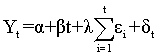(5)

is used where δt is stationary, βt is the trend component while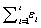is the random walk. βt=0 if we assume a without-trend regression. The series in equation will be stationary if λ=0. Regression is used to obtain the estimate of δt, that is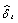, from which we compute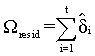(6)

The test statistic for KPSS test is then calculated as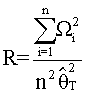(7)

where the spectral density function estimator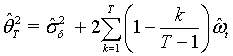(8)

is a linear combination of the variance estimator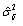and covariance estimator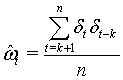(9)

The test turns to a prudential choice of T in equation above.

Review of phillip perron test: The Phillips Perron approach  applies a nonparametric correction to the standard ADF test statistic, allowing for more general dependence in the errors, including conditional heteroskedasticity. If there were strong concerns over heteroskedasticity in the ADF residuals this might influence an analyst to go for PP Phillip Perron test14. If the addition of lagged differences in ADF  did not remove serial correlation then this again might suggest PP as an alternative.

#### Empirical Results

Daily data on exchange rate of the Kenyan shilling against the dollar and the interbank lending rates are analysed in this section. The main aim is to establish if there exists any causality between the two rates. Granger causality is investigated in sub-section (5.1) while an ECM is built in sub-section (5.2) as follows:

Granger causality

The first step in granger causality analysis is to establish stationarity of the time series. A basic investigation of this property is by visual inspection of time plot. A time plot is plotted, represented in Figure 1 below, and by inspection the series is non stationary.

ACF Auto Correlation Function15 is conventionally used in time series analysis to inspect for stationarity. If the spikes tend to be constantly high close to a value of 1, the series is non stationary. Figure 2 below represents the respective ACFs of the two rates.

An inspection of the ACFs suggest non stationarity. The respective squares of the two series helps in the analysis of heteroskedasticity, which will determine the method used to investigate for causality. Nevertheless, mathematical tests such as KPSS, ADF and PP tests are necessary to ascertain non stationarity. ADF test output of the two series is presented in Table 1 below.

Exchange Rate Interbank Lending Rate
Dickey-Fuller Value -2.1937 -3.6382
Lag Order 14 14
P-value 0.4963 0.02897

Table 1: ADF Test output for exchange and interbank lending rates.

The ADF test tests the null of non stationarity. From the ADF test output above, an inspection of the p-values indicates that the null hypothesis is not rejected at 5% level of significance for the Dollar exchange rate. We reject the null hypothesis at 5% level of significance for the Interbank lending rate. Conventionally, p-value indicates the amount of evidence we have against the null. It is therefore concluded that the exchange rate is non stationary while the interbank lending rate might be stationary. However, the ADF test has two weaknesses, namely;

1. The model for an ADF test uses the differenced series.

2. It assumes that the residuals are independent and identically distributed.

These weakness call for the use of KPSS test. The KPSS test uses the series to test for non stationarity without differencing. The assumption on the distribution of the residuals is not required in this test. It is therefore a tentative alternative for stationarity test.

Contrary to the ADF test, the KPSS test tests for the null of stationarity. Therefore, from the output presented in Table 2 above, an inspection of the p-value calls for the rejection of the null hypothesis at 5% level of significance and conclude that the two series are not stationary. This is not in line with the results obtained from the ADF output in Table 1 above, where the interbank lending rate was established to be stationary. To ascertain these results, we apply the PP test. The results of this test is presented in the Table 3 below.

Exchange Rate Interbank Lending Rate
KPSS Level 5.0182 1.3153
Truncation Lag Parameter 13 13
P-value 0.01 0.01

Table 2: ADF Test output for exchange and interbank lending rates.

Exchange Rate Interbank Lending Rate
Dickey Fuller (Zα) -7.3937 -48.5564
Truncation Lag Parameter 9 9
P-value 0.6974 0.01

Table 3: ADF Test output for exchange and interbank lending rates.

Just like the ADF, the PP test tests the null of non stationarity. An inspection of the p-values indicates that the Interbank lending rate is stationary while the exchange rate is non stationary. This result is contrary to the results from KPSS test in Table 2 above, but in line with the results obtained from Table 1. This therefore calls for an informed judgement on whether to assume stationarity of the interbank lending rates. In this study, we will assume that the interbank lending rates are stationary and its only the exchange rate which is non stationary. This is because two of the three tests performed supports this judgement .

Following the above results, it is necessary to inspect whether the two series might be having a causal relationship. This can be investigated by superimposing the two series onto each other and checking whether their movements are similar.

Clearly from Figure 3 above, the two series have causal relationship.

The exchange rate series is thus differenced and checked for stationarity. The same tests are applied.

The null hypothesis is rejected at 5% level of significance. The exchange rate series is now stationary. Because of the weaknesses of the ADF test discussed above in Table 4, KPSS test is done and the results presented in Table 5 below.

Augmented Dickey-Fuller Test
Data:diff(Exchange)
Dickey-Fuller -14.2119
Lag order 14
p-value 0.01
Alternative hypothesis stationary

Table 4: ADF Test output for the differenced series of the exchange rate.

KPSS Test for Level Stationarity
data: diff(Exchange)
KPSS Level 0.0755
Truncation lag parameter 13
p-value 0.1

Table 5: KPSS Test output for the differenced series of exchange rate.

Phillips-Perron Unit Root Test
data: diff(Exchange)
Dickey-Fuller Z(alpha) -2765.71
Truncation lag parameter 9
p-value 0.01
alternative hypothesis stationary

Table 6: PP Test Output for the Differenced Series of Exchange Rate.

The results are same as for ADF test. We fail to reject the null hypothesis at 5% level of significance and conclude that the series is stationary. To ascertain these results, the PP test is done and resluts presented in Table 6 below.

This tests wraps up the unit root tests and we infer that the differenced series is stationary. It is therefore concluded that the exchange rate is I(1).

Estimation of lag value to be used in the estimation of causal relation between the two series follow. AIC is the commonly used procedure in the estimation. The output of the estimation is presented in the following Table 7.

Degrees of Freedom Sum of Squares RSS AIC
74156 10319
Diff(Exchange) 1 247.4 74403 10328

Table 7: Results for the AIC lag Estimation.

From the output, the second lag is the most appropriate for estimation. The model effects are therefore investigated and from Figure 4, it is clear that the model has a level effect except for tail values.

Since the model effects are level within the mean, the necessity of lag inclusion in the model is investigated. The results of this test is as shown in Table 8 below.

Granger causality test
Model 1 Y  Lags(Y, 1:1) + Lags(X, 1:1)
Model 2 Y  Lags(Y, 1:1)
Res.Df Df F Pr(>F) 1 3317 2 3318 -1 25.674 4.264e-07 ***

Table 8: Statistical Test output on lag inclusion in the model.

Y16 and X17 represents the interbank lending rate and the exchange rate, respectively. The null hypothesis of the saturated model is not rejected. Therefore, 3 sufficient evidence that the inclusion of the lagged values in causality estimation leads to the overall improvement in the model predictive ability. The coefficients for the granger causality model are presented in the Table 9 below.

Intercept X11 X12 X13
-7.9150651 0.1942408 0.1574291  -0.8701337

Table 9: Granger Causality Model Coefficients.

The third variable18 is found to be insignificant in the model, at 5% level of significance. The variable is dropped. A linear model is thus fit with the series itself and its second lagged value as follows (Table 10).

Intercept X11 X13
-7.9123922  0.1942063 -0.7914011

Table 10: A Linear Model with significant lagged values.

All the model parameters were found to be statistically significant at 5% level. From the output above, the granger causality model therefore becomes:

yt + 7.91239 = 0.19421xt -0.79140 xt-2 (10)

where yt represents the interbank lending rate while xt is the dollar exchange rate.

It therefore remains to check on the direction of the causality. The output is presented below Table 11.

F-statistic p-value
Exchange- Interbank 6.473963 0.00156268
Interbank- Exchange 2.992947 0.05027497

Table 11: Direction of the causality.

It is clear that exchange rates granger causes the interbanking lending rates. Therefore, movements in interbank lending rates are more likely to be caused by the movements in the exchange rates. However, interbank lending rate does not granger cause the exchange rate, as could have been expected.

Error correction model

Once the granger causality model discussed above has been built19, an ECM is easily built by considering the residuals of the model in equation above. ECM involves fitting a regression equation of the differenced series and the residuals of the fitted granger causality model. Due to the inclusion of the residuals, dynamic linear modeling is used. The fitted model will involve two main parts; the residual part which might be considered more stable than the differenced series part, hence the use of a dynamic linear model. The output of an estimated ECM is as shown in Table 12 below.

 Time series regression with "numeric" data: Start = 1, End = 3320 Call: dynlm(formula = Interbank3  Exchange4 + resid) Residuals: Min 1Q Median 3Q Max -4.5456 -0.6501 0.0874 0.5570 6.5569 Coefficients: Estimate Std. Error t value Pr(>|t|) (Intercept) 7.108730 0.021622 328.78 <2e-16*** Exchange4 -0.775746 0.055974 -13.86 <2e-16*** resid 1.000388 0.004744 210.85 <2e-16 *** --- Signif. codes: 0 ‘ ***’ 0.001 ‘ **’ 0.01 ‘ *’ 0.05 ‘ .’ 0.1 ‘ ’ 1 Residual standard error: 1.246 on 3317 degrees of freedom Multiple R-squared: 0.9308, Adjusted R-squared: 0.9308 F-statistic: 2.231e+04 on 2 and 3317 DF, p-value: < 2.2e-16

Table 12: An Estimation of an ECM for Exchange and Interbank Lending Rates.

All the parameters from the model are significant at 5% level. R2 value of 0.9308 shows that the overall model fits well to the data. The fitted ECM therefore becomes;

yt = 70108730 - 0.775746 Δ xt + 1.000388ᴧ (11)

where yt represents the interbank lending rate, xt the exchange rate while ᴧ denotes the error correction component.

#### Conclusion and Discussion

Contrary to time series theory, tests on interbank lending rates return stationarity. Nevertheless, the exchange rates seem to be consistently non stationary. The stationarity of the interbank lending rate can be attributed to the fact that;

• The interbank lending rates are controlled locally and are mainly set following fluctuation of world wide economic performance. The stability of interbank lending rates is mainly determined by the central bank.

• The exchange rate is normally controlled by the overall world wide economic performance. Its fluctuation is thus not influence locally by any country, i.e, it is not controlled monopolistically.

From the above arguements, we expect the interbank lending rates to be more stable of the two.

Results of the granger causality indicate that the exchange rates granger causes the interbank lending rates. Movements in exchange rates can be used as an indication of the most probable movement in interbank lending rate. The residual sum of squares from the granger causality model is very low, an indication that the model optimally explains the variations in the data. From the superimposed plot of the two series in Figure 2, it is expected that the exchange rate and the interbank lending rate granger causes each other. However, contrary to this intuition, the interbank lending rate does not granger cause the exchange rate. This can as well be attributed to the local nature of the interbank lending rate.

Finally, an ECM model is built and results presented in Table 11 in the above section. The model is presented in equation. From the output, the R2 value is 0.9308, an indication that the model well fits to the data. Further, the adjusted R2 value is 0.9308, same as the R2 value, meaning the model can as well perfectly fit to any other data with similar characteristics. Therefore, the model can be used for predictive purposes. This arguement is ascertained by the very small sum of squared residuals. The model can therefore be used to analyse data with similar characterisitcs as those used in the study. It can as well be adobted by institutions for their internal hedging and as a liquidity guard.

#### Recommendations

It is recommended that the same study be conducted with interbank lending rates being differenced to investigate whether their will be any change in the overall model. Also, an investigation on the stationarity of the interbank lending rates should be done to establish its cause and why it occurs. Further, a study on the tail values should be done to examine the cause of the tail clustering and its impact on the overall model. Given the granger causality model, it is recommended that a cointegration model be built to examine whether the two models differ from each other; and if so, examine the reasons for the difference.

1Generalized AutoRegressive Conditional Heteroskedasticity

2Granger Causality Model

3Error Correction Model

4At some level of significance

5Integration of order 0 or stationarity

6Integration of order 1

7London Interbank Offered Rate

8Morgan Stanley Capital International

9Banks

10Augmented Dickey Fuller test

11Less than 0.05 or 0.01 depending on the statistician

12Kwiatkowski Philips Schmidt Shin test

13If test statistic is with a trend

14Phillip Perron test

15Auto Correlation Function

16Interbank lending rate

17Exchange rate

18First lag of the exchange rate

19Equation

#### References

Select your language of interest to view the total content in your interested language

### Article Usage

• Total views: 8612
• [From(publication date):
December-2015 - Jul 19, 2019]
• Breakdown by view type
• HTML page views : 8421Can't read the image? click here to refresh